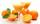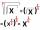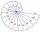Rotation

Right triangle with legs 14 cm and 20 cm rotate around longer leg.

Calculate the volume and surface area of the formed cone.

Result

S =  1689.5 cm2
V =  4105 cm3

Solution:Leave us a comment of example and its solution (i.e. if it is still somewhat unclear...):Be the first to comment!To solve this example are needed these knowledge from mathematics:

Tip: Our volume units converter will help you with converion of volume units.

Next similar examples:

1. Cone area and sideCalculate the surface area and volume of a rotating cone with a height of 1.25 dm and 17,8dm side.
2. Bottles of juiceHow many 2-liter bottles of juice need to buy if you want to transfer juice to 50 pitchers rotary cone shape with a diameter of 24 cm and base side length of 1.5 dm.
3. Ice cream in coneIn the ice cream cone with a diameter of 5.2 cm is 1.3 dl of ice cream. Calculate the depth of the cone.
4. GasholderThe gasholder has spherical shape with a diameter 20 m. How many m3 can hold in?
5. Cross-sections of a coneCone with base radius 16 cm and height 11 cm divide by parallel planes to base into three bodies. The planes divide the height of the cone into three equal parts. Determine the volume ratio of the maximum and minimum of the resulting body.
6. HoleIn the center of the cube with edge 14 cm we will drill cylinder shape hole. Volume of the hole must be 27% of the cube. What drill diameter should be chosen?
7. ConeCircular cone with height h = 29 dm and base radius r = 3 dm slice plane parallel to the base. Calculate distance of the cone vertex from this plane, if solids have the same volume.
8. SandpileAuto sprinkled with sand to an approximately conical shape. Workers wanted to determine the volume (amount of sand) and therefore measure the circumference of the base and the length of both sides of the cone (over the top). What is the volume of the san
9. CalculationHow much is sum of square root of six and the square root of 225?
10. Square rootsWhat is equal to the product of the square roots of 295936?
11. Theorem proveWe want to prove the sentence: If the natural number n is divisible by six, then n is divisible by three. From what assumption we started?
12. Surface areaThe volume of a cone is 1000 cm3 and the content area of the axis cut is 100 cm2. Calculate the surface area of the cone.
13. Truncated coneA truncated cone has a bases radiuses 40 cm and 10 cm and a height of 25 cm. Calculate its surface area and volume.
14. RootUse law of square roots roots: ?
15. Cylinder - A&VThe cylinder has a volume 1287. The base has a radius 10. What is the area of surface of the cylinder?
16. Cube volumeThe cube has a surface of 384 cm2. Calculate its volume.
17. Cylinder surface areaVolume of a cylinder whose height is equal to the radius of the base is 678.5 dm3. Calculate its surface area.